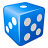# Revised Universal Soil Loss Equation LS Factor NoData areas

1827
1
10-15-2013 10:51 AMNew Contributor
Hi,

I was wondering if anyone had any ideas - For my dissertation I am trying to apply the revised universal soil loss equation to a
catchment using arcMAP. One of the parameters I have to calculate is the LS Factor (Slope Length/Slope Steepness Factor) which involves:

• Filling 5x5 DEM using the "fill" tool

• Using "Flow Accumulation" tool

• Using "Flow Direction" tool

• Using the depressionless DEM to calculate slope using the "slope" tool

I then use the following equation in raster calculator: Power("FlowAccumulation" * cell size/ 22.13,0.4) * Power((Sin("slope") / 0.0896,1.3)

This all looks fine and good, apart from the fact there are large noData gaps in my resulting layer.
I tried running the code written by Van Remortel et al 2004, but it produced exactly the same result! My DEM has no Nodata areas so I was wondering if anybody has any ideas what I can do to solve this problem?

Thanks! 🙂
Tags (3)